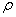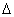## Resistance of a Wire

##### Resistance

Electrical charges can move easily in some materials (conductors) and less freely in others (insulators), as we learned previously. We describe a material’s ability to conduct electric charge as conductivity. Good conductors have high conductivities. The conductivity of a material depends on:

1. Density of free charges available to move
2. Mobility of those free charges

In similar fashion, we describe a material’s ability to resist the movement of electric charge using resistivity, symbolized with the Greek letter rho (). Resistivity is measured in ohm-meters, which are represented by the Greek letter omega multiplied by meters (•m). Both conductivity and resistivity are properties of a material.When an object is created out of a material, the material’s tendency to conduct electricity, or conductance, depends on the material’s conductivity as well as the material’s shape. For example, a hollow cylindrical pipe has a higher conductivity of water than a cylindrical pipe filled with cotton. However, shape of the pipe also plays a role. A very thick but short pipe can conduct lots of water, yet a very narrow, very long pipe can’t conduct as much water. Both geometry of the object and the object’s composition influence its conductance.

Focusing on an object’s ability to resist the flow of electrical charge, we find that objects made of high resistivity materials tend to impede electrical current flow and have a high resistance. Further, materials shaped into long, thin objects also increase an object’s electrical resistance. Finally, objects typically exhibit higher resistivities at higher temperatures. We take all of these factors together to describe an object’s resistance to the flow of electrical charge. Resistance is a functional property of an object that describes the object’s ability to impede the flow of charge through it. Units of resistance are ohms ().

For any given temperature, we can calculate an object’s electrical resistance, in ohms, using the following formula, which can be found on your reference table.In this formula, R is the resistance of the object, in ohms (), rho () is the resistivity of the material the object is made out of, in ohm*meters (•m), L is the length of the object, in meters, and A is the cross-sectional area of the object, in meters squared. Note that a table of material resistivities for a constant temperature is given to you on the reference table!

Let’s try a sample problem calculating the electrical resistance of an object:

Question: A 3.50-meter length of wire with a cross-sectional
area of 3.14 × 10–6 m2 at 20° Celsius has a resistance of 0.0625. Determine the resistivity of the wire and the material it is made out of.

Answer:## Introduction to Current Electricity##### Flow of ChargeElectric current is the flow of charge, much like water currents are the flow of water molecules. Water molecules tend to flow from areas of high gravitational potential energy to low gravitational potential energy. Electric currents flow from high electric potential to low electric potential. And the greater the difference between the high and low potential, the more current that flows!

In a majority of electric currents, the moving charges are negative electrons. However, due to historical reasons dating back to Ben Franklin, we say that conventional current flows in the direction positive charges would move. Although inconvenient, it’s fairly easy to keep straight if you just remember that the actual moving charges, the electrons, flow in a direction opposite that of the electric current. With this in mind, we can state that positive current flows from high potential to low potential, even though the charge carriers (electrons) actually flow from low to high potential.

Electric current (I) is measured in amperes (A), or amps, and can be calculated by finding the total amount of charge (q), in Coulombs, which passes a specific point in a given time (t). Electric current can therefore be calculated as:Question: A charge of 30 Coulombs passes through a 24-ohm resistor in 6.0 seconds. What is the current through the resistor?

Answer:##### Resistance

Electrical charges can move easily in some materials (conductors) and less freely in others (insulators), as we learned previously. We describe a material’s ability to conduct electric charge as conductivity. Good conductors have high conductivities. In similar fashion, we describe a material’s ability to resist the movement of electric charge using resistivity, symbolized with the Greek letter rho (). Resistivity is measured in ohm-meters, which are represented by the Greek letter omega multiplied by meters (•m). Both conductivity and resistivity are properties of a material.

Ball Lightning Sample: